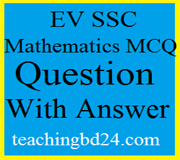# EV SSC MCQ Question Ans. Equations with One Variable

EV SSC MCQ Question Ans. Equations with One Variable. We have known in the previous class what equation is and learned its usage. We have learned the solution of simple equations with one variable and acquired knowledge thoroughly about the solution of simple equations by forming equations from real life problems. In this chapter, linear and quadratic equations and Identities have been discussed and their usages have been shown to solve the real life problems.

## EV SSC MCQ Question Ans. Equations with One Variable###There are two polynomials on two sides of the equal sign of an equation, or there may be zero on one side (mainly on the right-hand side). The degree of the variable of the polynomials on two sides may not be equal. Solving an equation, we get the number of values of the variable equal to the highest degree of that variable.

teachingbd24.com is such a website where you would get all kinds of necessary information regarding educational notes, suggestions and questions’ patterns of school, college and madrasahs. Particularly you will get here special notes of physics that will be immensely useful to both students and teachers. The builder of the website is Mr. Md. Shah Jamal Who has been serving for 30 years as Asst. Professor of BAF Shaheen College. He expects that this website will meet up all the needs of Bengali version learners /students. He has requested concerned both students and teachers to spread this website home and abroad.